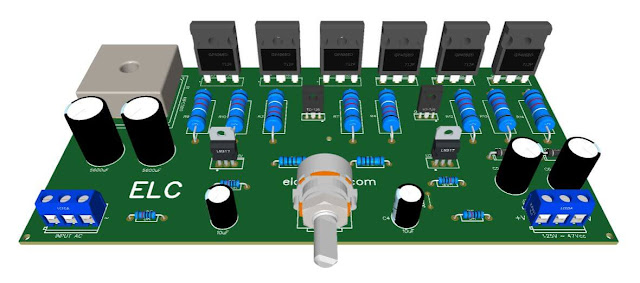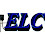## Wednesday, August 4, 2021Fig. 1 - Symmetrical Adjustable Power Supply 1.25V to 47V 10 Amps with Short Circuit Protection
This is a Symmetrical Adjustable Power Supply can vary its output voltage from 1.25V to 47V, based on the LM317HV Linear Voltage Regulator Integrated Circuit for positive voltage and the LM337HV for negative voltage, which together with the NPN transistors TIP 35C and the PNP transistor TIP36C provide a current of 10 amps.

This schematic was taken from our partner fvml.com.br, what we did was a small change in the supported current capacitance, you can check the original circuit here Click Here!

The LM317HV and LM337HV voltage regulators are adjustable 3-terminal voltage regulators capable of delivering currents of 1.5A or more over an output voltage range of 1.25V to 50V

The LM317HV and LM337HV offer overload protection such as current limiting, thermal overload protection, and safe-area protection, that make the device breakdown-proof. The overload protection circuit remains fully functional even if the setting terminal is disconnected.

Remember, we limit the maximum output voltage of the power supply to 47V, because the LM337HV negative voltage regulator supports a maximum of 50V, unlike the LM317HV which supports up to 60V.

## How the Circuit Works

After rectification and filtering, which are the first basic operations of the circuit, the total voltage coming from the transformer and rectified enters the first output block, the voltage regulator, which is controlled by the LMs Integrated Circuit and mirror image "Same function, just in a negative way".

R1 and R2 are 10 ohm resistors that have the function of Load Sensor, they receive the current flowing through the circuit, and while this current does not reach the current calculated across the resistors R1 and R2, the circuit behaves like a normal voltage regulator, because for small "calculated" currents there is no voltage drop across the Load Sensing resistor, so the Boosters Transistors TIP36C and TIP35C are not activated.

When the current in the circuit increases, the voltage across resistor R1 increases, when this voltage reaches about 0.6V "transistor turn-off voltage", the power stage is activated and current flows through it.

## The Protection Circuit

The output short circuit protection circuit is formed by transistors; Q1 BD140 PNP and Q2 BD139 NPN, each for an output bias voltage.

They regulate the maximum current "Calculated", which is fixed at 10 amps, and work together with 0.06ohm resistors R3 and R4 as a current sensing resistor, which is used to polarize transistors Q1 and Q2, so that, depending on the determined value, they limit the output current of the entire circuit according to a simple formula from Ohms Law, which is used to set this limiting current.

## Formula 1st Ohm's Law

The First Ohm's Law states that the potential difference between two points of a resistor is proportional to the electrical current established in it, and the ratio of electrical potential to electrical current is always constant for ohmic resistors. The formula is given by: V = R * I

V - Voltage or Electrical Potential
R - Electrical Resistance
I - Electrical Current

Armed with the knowledge of the ohms law, we can now calculate the values ​​of the Load Sense resistors, which activates the power step, and the bias resistors of the protection transistors, which is the Short Circuit protection circuit.

First, we have to know the current of the LM317hv Voltage Regulator, which according to the datasheet is 1.5 amps.

LM317HV & LM337HV = 1.5A
Let's calculate R1, knowing that the same calculation is done for R2. We know that Ohm's Law gives us the following expression:

V = R * I
V = The cutoff voltage of transistors Q3, Q4 & Q5, which follows the same principle for set Q6, Q7 & Q8, is 0.6V "Which is the Transistor cutoff region". Let's call Q3, Q4 & Q5 as a Qeq.

I = It is the current of the regulator CI1, let's put the working current of the CI1 at 300mA, which is equal to 0.3A, with this current we won't need to put a heatsink on it.

Then:

R1 = Vbe_Qeq / I_CI1
R1 = 0.6V / 0.3A
R1 = 2 ohms

### Protection Circuit Resistor Calculation

Likewise, we have to know the total current of the chosen source so that there is a cut in this region. Our source is for 10 Amps.

Power Supply = 10A
Let's calculate R3, knowing that the same calculation is done for R4. We know that Ohm's Law gives us the following expression:

V = R * I
V = The cutoff voltage of transistor Q1, which follows the same principle as for transistor Q2, is 0.6V "Which is the Transistor cutoff region".

I = It is the total current of PS, which is 10A.

Then:

R1 = Vbe_Q1 / I_ps
R1 = 0.6V / 10A
R1 = 0.06 ohms

### Power Transistors Current

Q3 + Q4 + Q5 = 25A + 25A + 25 = 75A

NOTE: Remembering that the power of TIP36C transistors is 125W, this means that it works with current from 25A to 5V, remember the formula above, P=V*I;

P = 5V * 25A = 125W.

For this circuit with a maximum voltage of 47V, and transistors with a maximum power of 125W, we look like this:

Pmax = V * I:
Imax = P / V => Imax = 125W / 47V => Imax = 2.66A
How are three transistors together Imax = 7.98A

And that's why our circuit uses three TIP36C transistors to achieve 10 amps at the output.

In Figure 2 we have the schematic diagram of the adjustable power supply circuit with short circuit protection, so those who accompany us already know this circuit very well, the difference is exactly the implantation of the symmetry of the circuit and the protection circuit, as we can see below.Fig. 2 - Symmetrical Adjustable Power Supply 1.25V to 47V 10 Amps with Short Circuit Protection

## The Power Transformer

The transformer must be symmetrical, i.e.: "3 wires". The transformer must be able to supply at least 10A at the output. The primary, "input voltage", must match the voltage in your area; 110V or 220Vac. The secondary, "output voltage" should be 36 - 0V - 36Vac.

## Component List

• Semiconductors
• U1 ....................... LM317HV Voltage Regulator
• U2 ....................... LM337HV Voltage Regulator
• Q1 ....................... PNP BD140 Transistor
• Q2 ....................... NPN BD139 Transistor
• Q3, Q4, Q5 ......... PNP TIP36C Power Transistor
• Q6, Q7, Q8 ......... NPN TIP35C Power Transistor
• D1 ...................... KBPC5010 - 50A Rectifier Bridge
• D2, D3 ............... 1N4007 Diode Rectifier

• Resistors
• R1, R2 ................ 2Ω / 2W  Resistor
• R3, R4 ................  0.06Ω / 5W Resistor
• R5, R6 ................ 5KΩ  / 1/8W Resistor
• R7, R8 ................ 120Ω / 1/8W Resistor
• R9, R10, R11 ...... 0.1Ω / 5W Resistor
• R12, R13, R14 .... 0.1Ω / 5W Resistor
• RV1 .................... 5KΩ Potentiometer

• Capacitors
• C1, C2 ................ 5600uF - 63V Electrolytic capacitor
• C3, C4 ................ 10uF - 63V Electrolytic capacitor
• C5, C6 ................ 1000uF - 63V Electrolytic Capacitor

• Others
• P1, P2 ................. Connector 3 screw terminal 5mm 3 Pins
• Others ................. Wires, Solders, pcb, etc.

We offer for download the necessary materials for those who want to assemble with PCI - Printed Circuit Board, the files in PNG, PDF and GERBER files for those who want to send for printing.

If you have any questions, suggestions or corrections, please leave them in the comments and we will answer them soon.

My Best Regards!!!

1.Parts list values of transistors and capacitor interchanged,compared with schematic diagram

1.Thank you very much for your feedback.
We have updated the list with the appropriate corrections.
Thank you!

2.1.Hi @BINHO
Welcome!
Regards!